Next: RL Circuit Up: Circuit Equations Previous: Circuit Equations

## RC Circuit

Consider the resistor R and capacitance C in the circuit loop in figure 2.1. Notice that there is no source.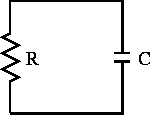Figure 2.1:  RC circuit.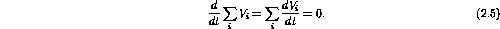When applied to our circuit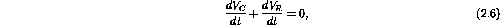where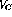is the voltage drop across the capacitor andis the voltage drop across the resistor.

The change in the voltage drop across the capacitor is given by our previous expression,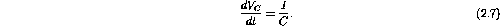The change in the voltage drop across the resistor can be obtained from Ohm's law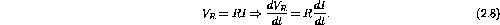Substituting these changes in voltage into Kirchoff's equation gives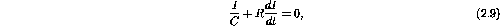where the current due to the flow of charge on or off the capacitor is the same as through the resistor.

Now we need some initial conditions. Notice that although the capacitor behaves as an open circuit to DC, current must flow to charge or discharge the capacitor. Lets take the case where the capacitor is initially charged and then the circuit is closed and the charge is allowed to drain off the capacitor (eg. closing a switch). The resulting current will flow through the resistor.

Solving for the current we obtain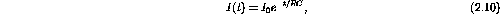where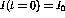is the initial current given by Ohm's law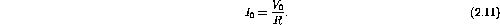Using a time dependent version of Ohm's law we can solve for the voltage across the resistor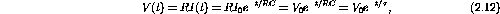where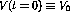is the initial voltage across the capacitor and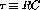is the commonly defined time constant of the decay. You should also be able to solve for the voltage across the capacitor and charge on the capacitor.

For the case of an applying voltage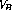being suddenly placed into the circuit (inserting a battery) the capacitor is initially not charged and the voltage across the capacitor is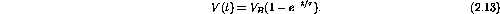In the first case, current and voltage exponentially decay away with time constantwhen the switch is closed. The charge flows off the capacitor and through the resistor. The energy initially stored in the capacitor is dissipated in the resistor.

In the second case the capacitor charges to a voltageuntil no current flows and hence the voltage drop across the resistor is zero. Energy from the battery is stored in the capacitor.

In both cases the characteristic RC time constant occurs. In general this is true of all resistor-capacitor combinations and will be important throughout the course.Next: RL Circuit Up: Circuit Equations Previous: Circuit Equations

Doug Gingrich
Tue Jul 13 16:55:15 EDT 1999